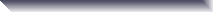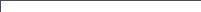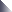SDL Component Suite - OpenArraysThe unit OPENARRAYS supports open arrays of dimensionality one to four. The unit contains many overloaded functions which allow you to manage and acccess integer and double precision open arrays. supports integer and double precision open arrays in one to four dimensions copying and filling of arrays matrix arithmetics (add, subtract, mulitply, accumulate, trace, cross products, etc.) determination of statistical parameters of the array elements discretization of values The unit OPENARRAYS is part of the SDL Component Suite. More details can be found in the online help pages.   What's new: Release 10.7   [Aug-24, 2020]   the function IsDichotomous tests whether the values of an array are dichotomous the function SortAndRemoveDuplicates sorts array values and removes duplicates the function SaveArrayAsImg allows to save a matrix as an image (BMP, PNG, JPG or clipboard) the functions ReadBinaryArrayHeader, LoadBinaryArray and SaveBinaryArray have been extended to support 3D and 4D matrices the functions RemoveColumn and RemoveRow delete columns and rows of an array the function CloneAndReverseArray clones an array and reverses the order of the elements the function FindInArray search for a value in an array (both integers and strings) the function SumOfSquaredDiffs calculates the sum of squared differences the function DistanceOfObjects calculates a multivariate distance the function FillArrayMasked allows to fill masked regions of a 2-dimensional matrix the functions SortArray and ShuffleArray sorts and randomizes one-dimensional arrays, respectively the function CalcHatMatrix calculates the hat matrix of a two-dimensional array the function EdgeVector calculates various parameters along the edge of a two-dimensional array the functions LoadBinaryArray and SaveBinaryArray load and save the 2D open arrays in binary form the function ReadBinaryArrayHeader reads the header of a binary array file BoolToNumArray converts a boolean array to a dichotomous numeric array the function FloodFillArray implements a flood filling algorithm for matrices DichotomizeArray extended to support more array types implemented an overloaded version of PercentileArray which supports 2-dim. arrays FindInSortedArray allows a quick search for array elements CloneArray extended to deal with single precision arrays InvertArray inverts boolean arrays MinMaxArray extended to TSingleArray MinArray extended to return the found positions of the minima for TIntArray and TInt2DArray MaxArray extended to support TInt3DArray and TInt4DArray PercentileArray calculates a quantile of the array values FrequDistOfArray calculates the frequency distribution of array values DichotomizeArray allows to create a boolean matrix from a numeric matrix FillArrayRix fills the array with a running index LoadArray loads data from files previously stored by StoreArray the functions CopyVectorToArrayColumn and CopyVectorToArrayRow copy a vector to an array the function EqualArrays compares two arrays bug fix: FillArray of boolean array did not work correctly Release 10.6   [Mar-09, 2018]  The unit OPENARRAYS is now available for all supported compilers, proving the following functions: AbsValArray, AccumulateArray, AddArrays, AddScalarToArray, CloneArray, CopyArray, CopyArrayColumn, CopyArrayRow, CountTrueCellsOfArray, CountValues, DiscretizeArray, FillArray, FillDiagArray, FillRandomNormal, FillRandomUniform, FlipDimArray, IncArray, MaxArray, MeanArray, MeanVarArray, MinArray, MinMaxArray, MultiplyArrayByScalar, MultiplyArrays, MultiplyArraysElemw, StdDevArray, StoreArray, SubtractArrays, TraceOfArray, TransposeArray, VectorCrossProduct, VectorDotProduct